## How to Calculate and Solve for Total Oil Pore Volume of Reservoir Fluid Flow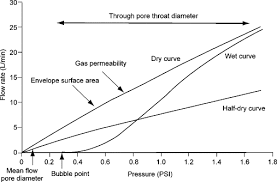The image above represents the total oil pore volume.

To compute for the total oil pore volume, four essential parameters are needed and these parameters are initial oil FVF (Boi), initial water saturation (Swi), oil in place (N) and gas cap ratio (m).

The formula for calculating the total oil pore volume:

(PV)o = NBoi(1 + m) / 1 + Swi

Where;

(PV)o = Total Oil Pore Volume
Boi = Initial Oil FVF
Swi = Initial Water Saturation
N = Oil in Place
m = Gas Cap Ratio

Let’s solve an example;
Find the total oil pore volume when the initial oil FVF is 9, the initial water saturation is 11, the oil in place is 21 and the gas cap ratio is 18.

This implies that;

Boi = Initial Oil FVF = 9
Swi = Initial Water Saturation = 11
N = Oil in Place = 21
m = Gas Cap Ratio = 18

(PV)o = NBoi(1 + m) / 1 + Swi
(PV)o = 21 x 9(1 + 18) / 1 + 11
(PV)o = 3591 / -10
(PV)o = -359.1

Therefore, the total oil pore volume is -359.1

## How to Calculate and Solve for Oil Saturation of Reservoir Fluid Flow | The Calculator Encyclopedia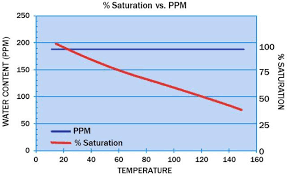The image above represents the oil saturation.

To compute for the oil saturation, five essential parameters are needed and these parameters are cumulative oil production (Np), oil in place (N), initial water saturation (Swi), initial oil FVF (Boi) and oil FVF (Bo).

The formula for calculating oil saturation:

S = [1 – Swi] [1 – Np / N] [Bo / Boi]

Where;

S = Oil Saturation
Np = Cumulative Oil Production
N = Oil in Place
Swi = Initial Water Saturation
Boi = Initial Oil FVF
Bo = Oil FVF

Let’s solve an example;
Given that the cumulative oil production is 22, oil in place is 8, initial water saturation is 16, initial oil FVF is 12 and the oil FVF is 7.
Find the oil saturation?

This implies that;

Np = Cumulative Oil Production = 22
N = Oil in Place = 8
Swi = Initial Water Saturation = 16
Boi = Initial Oil FVF = 12
Bo = Oil FVF = 7

S = [1 – Swi] [1 – Np / N] [Bo / Boi]
S = [1 – 16] [1 – 22/8] [7/12]
S = [1 – 16] [1 – 22/8] [0.583]
S = [1 – 16] [1 – 2.75] [0.583]
S = [1 – 16] [-1.75] [0.583]
S = [-15] [-1.75] [0.583]
S = 15.31

Therefore, the oil saturation is 15.31.

## How to Calculate and Solve for Cumulative GOR of Reservoir Fluid Flow | The Calculator Encyclopedia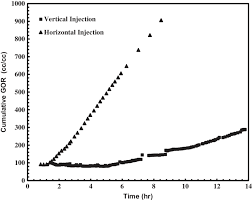The image above represents the cumulative GOR.

To compute for the cumulative GOR, two essential parameters are needed and these parameters are cumulative gas production (Gp) and cumulative oil production (Np).

The formula for calculating the cumulative GOR:

Rp = Gp / Np

Where;

Rp = Cumulative GOR
Gp = Cumulative Gas Production
Np = Cumulative Oil Production

Let’s solve an example;
Find the cumulative GOR with a cumulative gas production of 32 and a cumulative oil production of 44.

This implies that;

Gp = Cumulative Gas Production = 32
Np = Cumulative Oil Production = 44

Rp = Gp / Np
Rp = 32 / 44
Rp = 0.72

Therefore, the cumulative GOR is 0.72.

Calculating the Cumulative Gas Production when the Cumulative GOR and the Cumulative Oil Production is Given

Gp = Rp x Np

Where;

Gp = Cumulative Gas Production
Rp = Cumulative GOR
Np = Cumulative Oil Production

Let’s solve an example;
Find the cumulative gas production when the cumulative GOR is 56 and the cumulative oil production is 19.

This implies that;

Rp = Cumulative GOR = 56
Np = Cumulative Oil Production = 19

Gp = Rp x Np
Gp = 56 x 19
Gp = 1064

Therefore, the cumulative gas production is 1064.

## How to Calculate and Solve for Gas Initially in Place of a Reservoir Fluid Flow | The Calculator Encyclopedia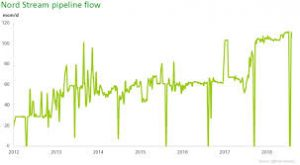The image above represents gas initially in place.

To compute for gas initially in place, five essential parameters are needed and these parameters are drainage area (A), initial gas FVF (Bgi), initial water saturation (Swi), porosity (φ) and thickness (h).

The formula for calculating gas initially in place:

G = 43560Ah φ (1 – Swi) / Bgi

Where;

G = Gas Initially in Place
A = Drainage Area
Bgi = Initial Gas FVF
Swi = Initial Water Saturation
φ = Porosity
h = Thickness

Let’s solve an example;
Find the gas initially in place with a drainage area of 44, initial gas FVF of 23, initial water saturation of 32, porosity of 18 and exponent of 14.

This implies that;

A = Drainage Area = 44
Bgi = Initial Gas FVF = 23
Swi = Initial Water Saturation = 32
φ = Porosity = 18
h = Thickness = 14

G = 43560Ah φ (1 – Swi) / Bgi
G = 43560 x 44 x 14 x 18 (1 – 32) / 23
G = 43560 x 44 x 14 x 18 (-31) / 23
G = -14972791680 / 23
G = -650990942.6

Therefore, the gas initially in place is -650990942.6

## How to Calculate and Solve for Gas Flow of Reservoir Fluid Flow | The Calculator Encyclopedia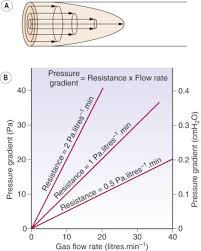The image above represents gas flow.

To compute for the gas flow, four essential parameters are needed and these parameters are Performance Co-efficient (C), Average Reservoir Pressure (Pr*), Flowing Bore Hole Pressure (Pwf*) and Exponent (n).

The formula for calculating the gas flow:

Qg = C(Pr*² – Pwf*²)n

Where;

Qg = Gas Flow
C = Performance Co-efficient
Pr* = Average Reservoir Pressure
Pwf* = Flowing Bore Hole Pressure
n = Exponent

Let’s solve an example;
Find the gas flow when a performance Co-efficient is 9, average reservoir pressure is 10, flowing bore hole pressure is 5 and an exponent of 20 is given.

This implies that;

C = Performance Co-efficient = 9
Pr* = Average Reservoir Pressure = 10
Pwf* = Flowing Bore Hole Pressure = 5
n = Exponent = 20

Qg = C(Pr*² – Pwf*²)n
Qg = 9(10² – 5²)20
Qg = 9(100 – 25)20
Qg = 9(75)20
Qg = 9(3.17e+37)
Qg = 2.85e+38

Therefore, the gas flow is 2.85e+38.

## How to Calculate and Solve for Total Compressibility of a Reservoir Fluid Flow | The Calculator Encyclopedia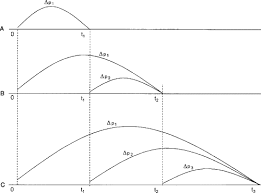The image above represents the total compressibility.

To compute for the total compressibility, seven essential parameters are needed and these parameters are Water compressibility (Cw), Oil compressibility (Co), Gas compressibility (Cg), Rock compressibility (Cr), water saturation (Sw), Oil saturation (So) and Gas saturation (Sg).

The formula for calculating the total compressibility:

CT = CoSo + CgSg + CwSw + Cr

Where;

CT = Total Compressibility
Cw = Water Compressibility
Co = Oil Compressibility
Cg = Gas Compressibility
Cr = Rock Compressibility
Sw = Water Saturation
So = Oil Saturation
Sg = Gas Saturation

Let’s solve an example;
With a given value of 13 as water compressibility, 17 as oil compressibility, 19 as gas compressibility, 25 as rock compressibility, 24 as water saturation, 29 as oil saturation and 21 as gas saturation.
Find the total compressibility?

This implies that;

Cw = Water Compressibility = 13
Co = Oil Compressibility = 17
Cg = Gas Compressibility = 19
Cr = Rock Compressibility = 25
Sw = Water Saturation = 24
So = Oil Saturation = 29
Sg = Gas Saturation = 21

CT = CoSo + CgSg + CwSw + Cr
CT = (17 x 29) + (19 x 21) + (13 x 24) + 25
CT = 493 + 399 + 312 + 25
CT = 1229

Therefore, the total compressibility is 1229 psi.

## How to Calculate and Solve for the Water – Oil Ratio of a Reservoir Fluid Flow | The Calculator Encyclopedia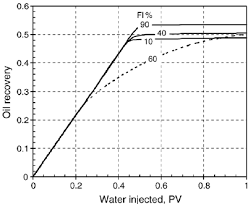The above image represents Water – Oil Ratio.

To compute for the water – oil ratio, six essential parameters are needed and these parameters are o)Oil Viscosity, (μw)Water Viscosity, (krw)Relative Permeability to Water, (kro)Relative Permeability to Oil, (Bw)Water Formation Volume Factor and (Bo)Oil Formation Volume Factor.

The formula for calculating the water – oil ratio:

WOR = [krw / kro] [μoBo  / μwBw ]

Where;

WOR = Water Oil Ratio
μo = Oil Viscosity
μw = Water Viscosity
krw = Relative Permeability to Water
kro = Relative Permeability to Oil
Bw = Water Formation Volume Factor
Bo = Oil Formation Volume Factor

Let’s solve an example;
Find the water – oil ratio when the oil viscosity is 9, water viscosity is 11 with a relative permeability to water of 21, relative permeability to oil of 7 and a water formation volume factor of 14 with oil formation volume factor of 27.

This implies that;

μo = Oil Viscosity = 9
μw = Water Viscosity = 11
krw = Relative Permeability to Water = 21
kro = Relative Permeability to Oil = 7
Bw = Water Formation Volume Factor = 14
Bo = Oil Formation Volume Factor = 27

WOR = [krw / kro] [μoBo  / μwBw ]
WOR = [21/7] [9 x 27 /11 x 14]
WOR = [21/7] [243/154]
WOR = [21/7] [1.5779]
WOR =  [1.5779]
WOR = 4.73

Therefore, the Water – Oil Ratio is 4.73.

## How to Calculate and Solve for the Average Pressure of a Reservoir Fluid Flow | The Calculator Encyclopedia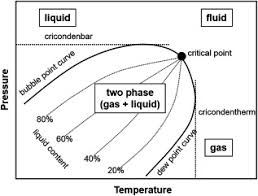The above image represents the reservoir fluid.

To compute for the reservoir fluid, two essential parameters are needed and these parameters are External pressure (Peand Flowing Bottom-Hole Pressure (Pwf).

The formula for calculating the average pressure of the reservoir fluid:

P* = √[Pe² + Pwf² / 2]

Where;

P* = Average Pressure
Pe = External Pressure
Pwf = Flowing Bottom-Hole Pressure

Let’s solve an example;
Find the average pressure of a reservoir fluid with an external pressure of 19 and Flowing bottom-hole pressure is 15.

This implies that;

Pe = External Pressure = 19
Pwf = Flowing Bottom-Hole Pressure = 15

P* = √[Pe² + Pwf² / 2]
P* = √[19² + 15² / 2]
P* = √[361 + 225 / 2]
P* = √[586 / 2]
P* = √
P* = 17.117

Therefore, the average pressure is 17.117 psi;.

Calculating the External Pressure when the Average Pressure and the Flowing Button-Hole Pressure is Given.

Pe = √[[P* x 2]² – Pwf²]

Where;

Pe = External Pressure
P* = Average Pressure
Pwf = Flowing Bottom-Hole Pressure

Let’s solve an example;
Find the external pressure of a reservoir fluid with an average pressure of 24 and Flowing bottom-hole pressure is 16.

This implies that;

P* = Average Pressure = 24
Pwf = Flowing Bottom-Hole Pressure = 16

Pe = √[[P* x 2]² – Pwf²]
Pe = √[[24 x 2]² – 16²]
Pe = √
Pe = 45.25

Therefore, the external pressure is 45.25.

## How to Calculate and Solve for Radial Flow Rate in Reservoir Fluid Flow | The Calculator Encyclopedia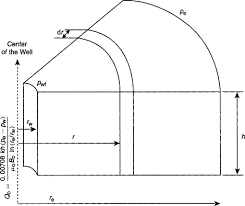The image above represents the radial flow rate.

To compute for the radial flow rate, eight essential parameters are needed and these parameters are External Pressure (Pe), Flowing Bottom-Hole Pressure (Pwf), Formation Thickness (h), Oil Viscosity (μo ), Permeability (k), Oil Formation Volume Factor (Bo), Drainage Radius (reand Well Bore Radius (rw ).

The formula for calculating the radial flow rate:

Qo = 0.00708kh[Pe – Pwf] / μo Bo In[re / rw]

Where;

Pe = External Pressure
Pwf = Flowing Bottom-Hole Pressure
h = Formation Thickness
μo = Oil Viscosity
k = Permeability
Bo = Oil Formation Volume Factor

Let’s solve an example;
Find the radial flow rate when the External Pressure is 14, Flowing Bottom-Hole Pressure is 21, Formation Thickness is 7, Oil Viscosity is 35, Permeability is 50, Oil Formation Volume Factor is 13, Drainage Radius is 26 and Well Bore Radius is 15.

This implies that;

Pe = External Pressure = 14
Pwf = Flowing Bottom-Hole Pressure = 21
h = Formation Thickness = 7
μo = Oil Viscosity = 35
k = Permeability = 50
Bo = Oil Formation Volume Factor = 13
re = Drainage Radius = 26
rw = Well Bore Radius = 15

Qo = 0.00708kh[Pe – Pwf] / μo Bo In[re / rw]
Qo = 0.00708 x 50 x 7 [14 – 21] / 35 x 13 In[26 / 15]
Qo = 0.00708 x 50 x 7 [-7] / 35 x 13 In[26 / 15]
Qo = 0.00708 x 50 x 7 [-7] / 35 x 13 In[1.73]
Qo = 0.00708 x 50 x 7 [-7] /35 x 13 x 0.55
Qo = 2.478 [-7] / 35 x 13 x 0.55
Qo = -17.346 / 250.27
Qo = -0.069

Therefore, the radial flow rate is -0.069 STB/day.

## How to Calculate and Solve for Linear Flow Rate in Reservoir Fluid Flow | The Calculator Encyclopedia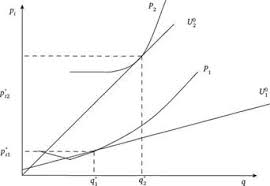The image above represents the linear flow rate.

To compute for the linear flow rate, four parameters are needed and these parameters are Initial pressure (P1), Final Pressure (P2), Thickness (h) and Permeability (k).

The formula for calculating linear flow rate:

q = 0.001127kh[P1 – P2]

Where:
q = Linear Flow Rate
P1 = Initial Pressure
P2 = Final Pressure
h = Thickness
k = Permeability

Let’s solve an example;
Find the linear flow rate when the initial pressure is 12, final pressure is 22, thickness is 18 and permeability is 44.

This implies that;
P1 = Initial Pressure = 12
P2 = Final Pressure = 22
h = Thickness = 18
k = Permeability = 44

q = 0.001127kh [P1 – P2]
q = 0.001127 x 44 x 18 [12 – 22]
q = 0.001127 x 44 x 18 [-10]
q = 0.892584 [-10]
q = -8.92584

Therefore, the linear flow rate is -8.92584 bbl/day.

Calculating Permeability when the linear flow rate, Initial Pressure, Final Pressure and Thickness is Given.

k = q / 0.001127h (p1 – p2)

Where;
k = Permeability
q = Linear Flow Rate
P1 = Initial Pressure
P2 = Final Pressure
h = Thickness

Let’s solve an example;
Find the permeability when the initial pressure is 34, final pressure is 24, thickness is 12 and linear flow rate is 50.

This implies that;
q = Linear Flow Rate = 50
P1 = Initial Pressure = 34
P2 = Final Pressure = 24
h = Thickness = 12

k = q / 0.001127h (p1 – p2)
k = 50 / 0.001127h (34 – 24)
k = 50 / 0.001127h (10)
k = 50 / 0.01127
k = 4436.5

Therefore, the permeability is 4436.5.